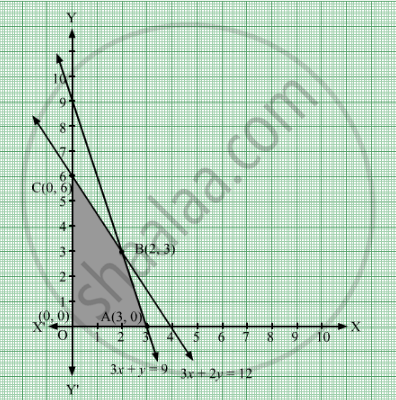# A Manufacturer Produces Two Products a and B. Both the Products Are Processed on Two Different Machines.Find the Production Level per Day for Maximum Profit Graphically. - Mathematics

Sum

A manufacturer produces two products and B. Both the products are processed on two different machines. The available capacity of first machine is 12 hours and that of second machine is 9 hours per day. Each unit of product A requires 3 hours on both machines and each unit of product B requires 2 hours on first machine and 1 hour on second machine. Each unit of product A is sold at ₹7 profit and that of at a profit of ₹4. Find the production level per day for maximum profit graphically.

#### Solution

Let x units of product A and y units of product B be manufactured by the manufacturer per day.
It is given that one unit of product A requires 3 hours of processing time on first machine, while one unit of product B requires 2 hours of processing time on first machine. It is also given that first machine is available for 12 hours per day.
∴ 3x + 2y ≤ 12
Also, one unit of product A requires 3 hours of processing time on second machine, while one unit of product B requires 1 hour of processing time on second machine. It is also given that second machine is available for 9 hours per day.
∴ 3x + y ≤ 9
The profits on one unit each of product A and product B is ₹ 7 and ₹ 4, respectively.
So, the objective function is given by Z = ₹ (7x + 4y).
Therefore, the mathematical formulation of the given linear programming problem can be stated as:
Maximize Z = 7x + 4
Subject to the constraints
3x + 2y ≤ 12         .....(1)
3x + y ≤ 9             .....(2)
x ≥ 0, y ≥ 0           .....(3)
The feasible region determined by constraints (1) to (3) is graphically represented as:Here, it is seen that OABCO is the feasible region and it is bounded. The values of Z at the corner points of the feasible region are represented in tabular form as:

 Corner Point Z = 7x + 4y O(0, 0) Z = 7 × 0 + 4 × 0 = 0 A(3, 0) Z = 7 × 3 + 4 × 0 = 21 B(2, 3) Z = 7 × 2 + 4 × 3 = 26 C(0, 6) Z = 7 × 0 + 4 × 6 = 24

The maximum value of Z is 26, which is obtained at x = 2 and y = 3.
Thus, 2 units of product A and 3 units of product B should be manufactured by the manufacturer per day in order to maximize the profit.
Also, the maximum daily profit of the manufacturer is ₹ 26.

Concept: Graphical Method of Solving Linear Programming Problems
Is there an error in this question or solution?

#### APPEARS IN

RD Sharma Class 12 Maths
Chapter 30 Linear programming
Exercise 30.4 | Q 53 | Page 57